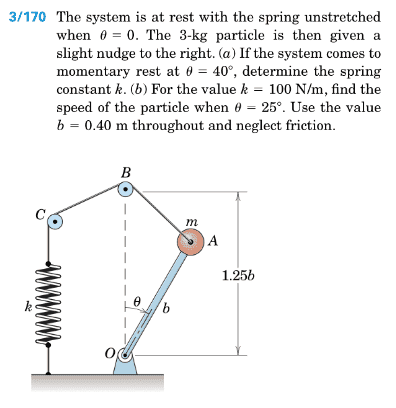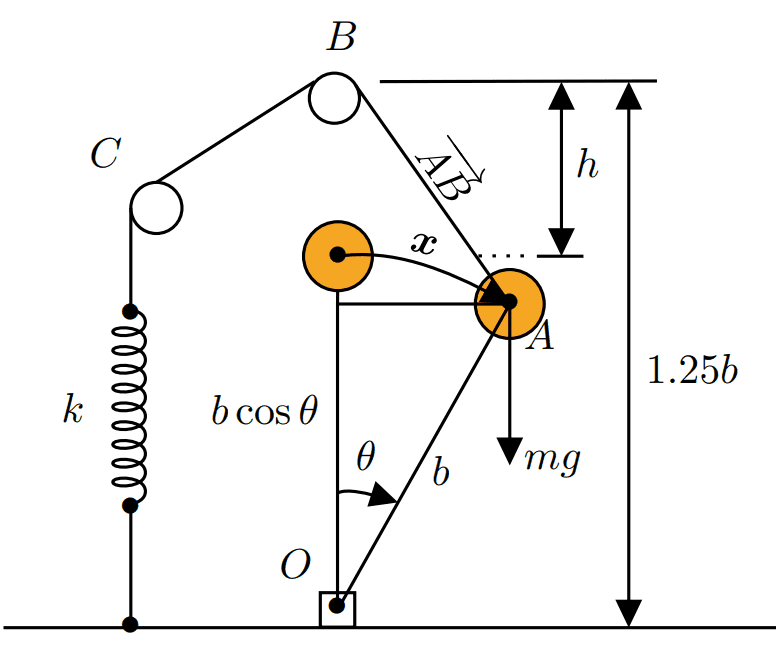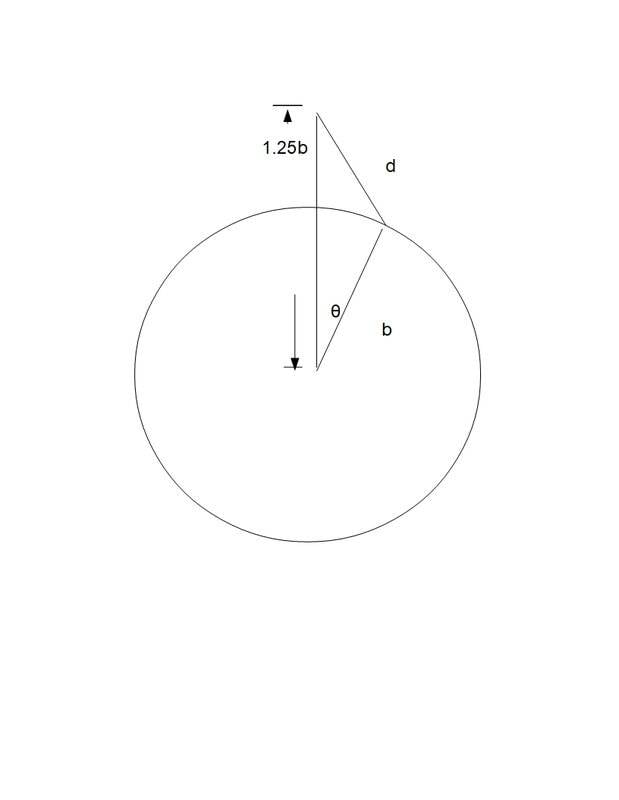# Lagrangian mechanics, system of a spring and a pendulum

TimmyD1
Homework Statement:
System of spring and pendulum
Relevant Equations:
L=T-VHello! I have some problem getting the correct answer for (b).
My FBD:For part (a) my lagrangian is
$$L=T-V\iff L=\frac{1}{2}m(b\dot{\theta})^2+mg(b-b\cos\theta)-\frac{1}{2}k\boldsymbol{x}^2,\ where\ \boldsymbol{x}=\sqrt{(1.25b-b)^2+(b\sin\theta)^2}-(1.25b-0.25b)$$

Hence my equation of motion become

$$\frac{1}{2}mb^2\ddot{\theta}+mg(b-b\cos\theta)-\frac{1}{2}k\boldsymbol{x}^2=0$$

solving this gives me the spring coefficient $$k=111.92\ N/m$$.

for part (b):

when $$\theta=25^{\circ}\ and\ k=100\ N/m$$ then

$$\frac{1}{2}mb^2\dot{\theta}\frac{d\dot{\theta}}{d\theta}+mg(b-b\cos\theta)-\frac{1}{2}k\boldsymbol{x}^2=0\iff mb^2\dot{\theta}\frac{d\dot{\theta}}{d\theta}+2mgb(1-\cos\theta)-k\boldsymbol{x}^2=0\iff \dot{\theta} d\dot{\theta} = \Big[\frac{k\boldsymbol{x}^2}{mb^2}-\frac{2g}{b}(1-\cos\theta)\Big]\ d\theta$$

$$\int_0^{\dot{\theta}} \dot{\theta} d\dot{\theta} = \int_0^{\frac{25\pi}{180}}\underbrace{\Big[\frac{k\boldsymbol{x}^2}{mb^2}-\frac{2g}{b}(1-\cos\theta)\Big]}_{-1.7001}\ d\theta\Longrightarrow \frac{\dot{\theta}^2}{2}=0.74384\iff \dot{\theta}=\sqrt{2\cdot 0.74384}=1.2197\ rad/s\Longrightarrow v=r\omega=b\dot{\theta}=0.4\cdot1.2197\approx 0.48788\ m/s$$

The answer should be v = 0.522 m/s but I can't seem to get it right, would really appreciate it if anyone could help me out!

Why do you want to use the Lagrangian formalism here? You don't need to determine the equations of motion, you can just conserve mechanical energy in a single equation.

TimmyD1
The exercise is to use lagrangian formalism, I have solved it using energy principle but using the lagrangian i ran into problems.

I don't think your spring potential energy is correct. I assume the ##x## on your diagram labelled next to the curved line segment is the arc length traversed by the mass (in which case I have no idea why you use Pythagorean theorem... it should just be ##x = b\theta##, not ##x=\sqrt{(1.25b-b)^2+(b\sin\theta)^2}##), however this length isn't relevant to the extension of the spring.

(N.B. Also you should not use bold font ##\mathbf{x}## since it is not a vector; just call it ##x##)

The distance you actually want is the magnitude of the vector ##|\overrightarrow{AB}|##,$$|\overrightarrow{AB}| = b\sqrt{\frac{41}{16} - 2.5 \cos{\theta}}$$from this you subtract off ##0.25b## to get the extension ##\delta## of the spring,$$\delta = |\overrightarrow{AB}| - 0.25b = b \left( \sqrt{\frac{41}{16} - 2.5 \cos{\theta}} - 0.25 \right)$$Then the Lagrangian should read$$L=\frac{1}{2}mb^2 \dot{\theta}^2+mgb(1-\cos\theta)-\frac{1}{2}k b^2 \left( \sqrt{\frac{41}{16} - 2.5 \cos{\theta}} - 0.25 \right)^2$$

TimmyD1
I ran into the same integral using the lagrangian you defined

Really? Because you defined some parameter ##x## as$$x =\sqrt{(1.25b-b)^2+(b\sin\theta)^2}-(1.25b-0.25b)$$which doesn't seem to make sense geometrically and doesn't equal my extension ##\delta##. When you plug ##\theta = 25^o## into your ##x##, you get a negative number, which should be a sign that something is off.

TimmyD1
Sorry it's a typo that I just realized,

$$x=\sqrt{(1.25b-b\cos\theta)^2+(b\sin\theta)^2}-0.25b$$

if we can ignore the notation and geometric point of view for now, it just bothers me why I can't get the same answer using lagrangian. I think that using $$v=r\omega$$ relation messes it up, because I can't seem to find the error.

•etotheipi
Sorry it's a typo that I just realized,

$$x=\sqrt{(1.25b-b\cos\theta)^2+(b\sin\theta)^2}-0.25b$$

That's the correct extension, yesBut I'll note that what you have labelled ##x## in your diagram is not this length; the ##x## in your diagram equals ##b\theta##, i.e. the length of the curved line segment. This expression you have just written is actually ##|\overrightarrow{AB}| - 0.25b##.

With that cleared up, now let's derive the equations of motion for the Lagrangian:$$L=\frac{1}{2}m(b\dot{\theta})^2+mg(b-b\cos\theta)-\frac{1}{2}k(\sqrt{(1.25b-b\cos\theta)^2+(b\sin\theta)^2}-0.25b)^2$$We have that$$\frac{\partial L}{\partial \dot{\theta}} = mb^2 \dot{\theta} \implies \frac{d}{dt} \frac{\partial L}{\partial \dot{\theta}} = mb^2 \ddot{\theta}$$ $$\frac{\partial L}{\partial \theta} = mgb\sin{\theta} - \frac{k(\sqrt{(1.25b-b\cos\theta)^2+(b\sin\theta)^2}-0.25b)}{2\sqrt{(1.25b-b\cos\theta)^2+(b\sin\theta)^2}} \left( 2b\sin{\theta} (1.25b - b\cos{\theta}) + 2b^2\sin{\theta} \cos{\theta}\right)$$where I haven't simplified that final expression and hope I haven't made a mistake somewhere. To find equation of motion you need to solve the ODE resulting from $$\frac{d}{dt} \frac{\partial L}{\partial \dot{\theta}} = \frac{\partial L}{\partial \theta}$$

I think that using $$v=r\omega$$ relation messes it up, because I can't seem to find the error.

No your kinetic energy term is completely fine. You can get it by working in polars, e.g. ##T = \frac{1}{2}m \vec{v} \cdot \vec{v} = \frac{1}{2}m (r\dot{\theta} \hat{\theta}) \cdot (r\dot{\theta} \hat{\theta}) = \frac{1}{2}mr^2 \dot{\theta}^2##.

•Abhishek11235
TimmyD1
So the acceleration would simply be

$$\ddot{\theta}=\frac{\dfrac{\partial L}{\partial \theta}}{mb^2}$$

now I would assume to integrate the expression to get velocity but not sure what the bounds would be

$$\int_0^{\dot{\theta}}\ddot{\theta}d\ddot{\theta}=\int_0^{\frac{25\pi}{180}}\frac{\dfrac{\partial L}{\partial \theta}}{mb^2}\ d\theta\ ?$$

$$\int_0^{\dot{\theta}}\ddot{\theta}d\ddot{\theta}=\int_0^{\frac{25\pi}{180}}\frac{\dfrac{\partial L}{\partial \theta}}{mb^2}\ d\theta\ ?$$

If you have the equation$$\ddot{\theta} = \frac{1}{mb^2} \frac{\partial L}{\partial \theta}$$then both sides must be integrated with respect to the same variable*; but you integrated the LHS w.r.t. ##\ddot{\theta}## and the RHS w.r.t. ##\theta##, which is an incorrect step.

*(As an example, remember that if ##f=g##, then ##\Gamma(f) = \Gamma(g)##. You can define ##\Gamma(f) := \int_{x_1}^{x_2} f(x) dx##. It follows that you can integrate both sides w.r.t. the same variable)

What you might think of is to try$$c+\dot{\theta} = \int \ddot{\theta} dt = \frac{1}{mb^2} \int \frac{\partial L}{\partial \theta} dt$$however you can't do this because the RHS depends on ##\theta##. This way won't work.

Last edited by a moderator:
TimmyD1
So with the help of MATLAB, I've found that

$$\frac{1}{mb^2}\int\frac{\partial L}{\partial \theta}dt\approx 0.83719t+C$$

What next!?

No you can't do it, because ##\frac{\partial L}{\partial \theta}## is a function of ##\theta##. How are you going to integrate with respect to time? You will require another approach.

To reiterate, you want to solve the (unsimplified) differential equation in ##\theta(t)##,$$mb^2 \frac{d^2 \theta}{dt^2} = mgb\sin{\theta} - \frac{k(\sqrt{(1.25b-b\cos\theta)^2+(b\sin\theta)^2}-0.25b)}{2\sqrt{(1.25b-b\cos\theta)^2+(b\sin\theta)^2}} \left( 2b\sin{\theta} (1.25b - b\cos{\theta}) + 2b^2\sin{\theta} \cos{\theta}\right)$$

TimmyD1
Alright! Before I tackle this, do we have any initial conditions?

You will need to specify a finite ##\theta(0) = \theta_0##. Because if ##\theta(0) = 0## then the system will remain at rest indefinitely. You can also set ##\dot{\theta}(0) = 0##.

Once you've done that, and checked that I didn't make a mistake in ##\partial L/\partial \theta##, then Mathematica's probably the way to go...

Homework Helper
Gold Member
So the acceleration would simply be
$$\ddot{\theta}=\frac{\dfrac{\partial L}{\partial \theta}}{mb^2}$$
A trick is to multiply both sides by ##\dot \theta##.
Each side can then be expressed as the time derivative of some expression (a different expression for each side).

•Abhishek11235 and etotheipi
Homework Helper
Gold Member
A trick is to multiply both sides by ##\dot \theta##.
It helps to note that for your particular Lagrangian, ##\frac{\partial L}{\partial \theta} = -\frac{\partial V}{\partial \theta}##.

As @etotheipi noted, you obtain a first-order differential equation in ##\dot \theta## and ##\theta##.
It expresses conservation of _____________ (fill in the blank).

•etotheipi
TimmyD1
I could not solve the differential equation and I think finding the acceleration of the system will be enough for me!

Amazing guidance by the way!

TimmyD1
It helps to note that for your particular Lagrangian, ##\frac{\partial L}{\partial \theta} = -\frac{\partial V}{\partial \theta}##.

As @etotheipi noted, you obtain a first-order differential equation in ##\dot \theta## and ##\theta##.
It expresses conservation of _____________ (fill in the blank).

$$\dfrac{d}{dt}\dfrac{\partial L}{\partial \dot{\theta}}=mb^2\ddot{\theta}=\dfrac{\partial L}{\partial\theta}$$ Multiply both sides with $$\dot{\theta}$$

gives

$$\frac{1}{2}mb^2\frac{d\dot{\theta}^2}{dt}=\dfrac{\partial L}{\partial \theta}\dot{\theta}=-\frac{dV}{d\theta}\dot{\theta}=-\frac{dV}{dt}$$ such that $$\dfrac{d}{dt}\Big(\frac{1}{2}mb^2\dot{\theta}+V\Big)=0$$ which gives us a constant.

$$\dfrac{\partial L}{\partial \theta}\dot{\theta}=-\frac{dV}{d\theta}\dot{\theta}=-\frac{dV}{dt}$$
I think you should have a partial here instead,$$\dfrac{\partial L}{\partial \theta}\dot{\theta}=-\frac{\partial V}{\partial \theta}\dot{\theta}=-\frac{dV}{dt}$$but that's not such a big deal$$\dfrac{d}{dt}\Big(\frac{1}{2}mb^2\dot{\theta}+V\Big)=0$$
You miss a square (probably just typo, since you had it in previous line)$$\dfrac{d}{dt} \left(\frac{1}{2}mb^2\dot{\theta}^2+V \right)=0$$And ##\frac{1}{2}mb^2\dot{\theta}^2+V## is a special constant, it is the energy ##E##!

TimmyD1
Another way of looking at the solution is to study what is usually called the energy function

$$h(q_1...\dot{q}_n,\dot{q}_1...\dot{q}_n,t)=\sum_j\dot{q}_j\frac{\partial L}{\partial \dot{q}_j}-L$$

The energy function is derived as one term from the expression for

$$\frac{dL}{dt}$$

when replacing (from Euler Lagrange :)

$$\frac{\partial L}{\partial q_j}=\frac{d}{dt}\frac{\partial L}{\partial\dot{q}_j}$$

The energy function is identical with the Hamiltonian (albeit in disguised form), cf.

$$H(q_j,p_j,t)$$

If L does not depend explicitly on time, but only implicitly, ie the second term ##\dfrac{\partial L}{\partial t}=0## in the derivation, ##h## is conserved. We can set it to an arbitrary value (the potential is arbitrarily determined), e.g. 0.

For our specific case, the following applies:

$$h(\theta,\dot{\theta})=\dot{\theta}\frac{\partial L}{\partial \dot{\theta}}-L=0$$

With inserted expressions
$$T=\frac{mb^2\dot{\theta}^2}{2},\ V=\frac{kx^2}{2}-mgb(1-cos\theta)\leadsto mb^2\dot{\theta}^2-(\frac{1}{2}mb^2\dot{\theta}^2-V)=0$$

one can add that the last equation is the same as ##T + V = 0##, which means that we have shown that the energy of the system, the sum of the kinetic and the potential energy, must be constant. A constant we can choose arbitrarily through the potential.

Fred Wright
1. In the diagram below##d^2=(1.25b)^2+ b^2 - 2(1.25b)b\cos(\theta)##

##d(\theta)=b\sqrt (2.5625-2.5\cos(\theta))##

The spring extension is ##d(\theta)-.25b## and therefore the spring potential energy is ##\frac{1}{2}k(b\sqrt (2.5625-2.5\cos(\theta)) - .25b)^2##

2. You can show, using the chain rule,
##\ddot{\theta}=\dot{\theta}\frac{d\dot{\theta}}{d\theta}##

From this if you have,$$\ddot{\theta}=f(\theta)$$
you can integrate to get$$\int_{\dot{\theta_{init}}}^{\dot{\theta_{final}}}\dot{\theta}d\dot{\theta}=\int_{\theta_{init}}^{\theta_{final}}f(\theta)d\theta$$

Last edited: